# Sudoku

## Overview

The rules of Sudoku are simple: fill in a 9x9 grid with the digits 1 through 9, such that every row, column, and 3x3 tile contains each digit exactly once. Here's a Sudoku puzzle and solution: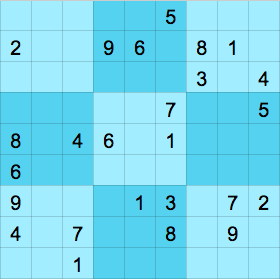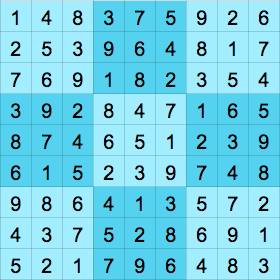The Sudoku Sheets add-on is available here. Click on the "FREE" button to install, which makes it available to Google Sheets whenever you're logged in to Google.

After you grant the necessary permissions, you're ready to create and solve Sudokus.

## Creating Sudokus

To create a Sudoku, select Add-ons -> Sudoku Sheets -> Generate sudoku: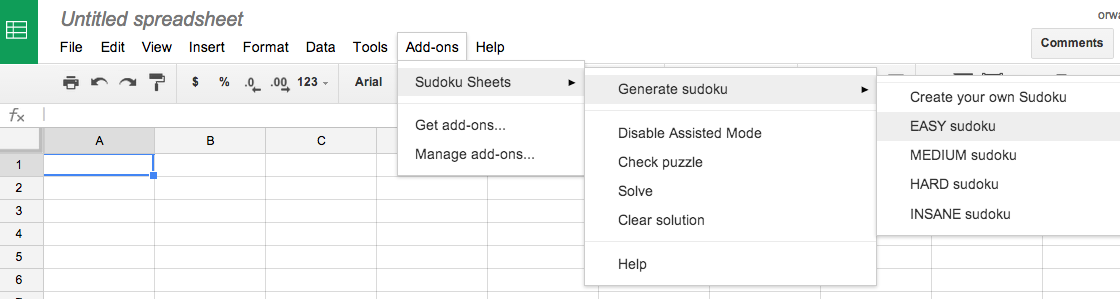If you want to solve a Sudoku generated elsewhere, select "Create your own Sudoku".

## Solving Sudokus

To solve the Sudoku, just select a cell and enter a number.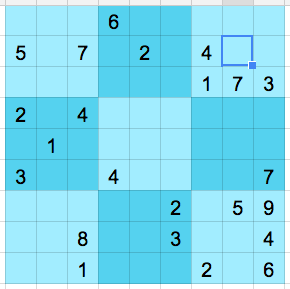By default, Sudoku Sheets run in Assisted Mode, which flags mistakes once you select another cell in the spreadsheet: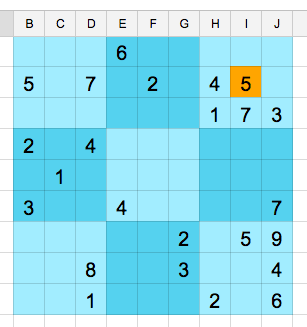You can turn off Assisted Mode via the menu, in which case you can work toward a complete grid and then select "Check Solution".

And if you give up, select "Solve Sudoku":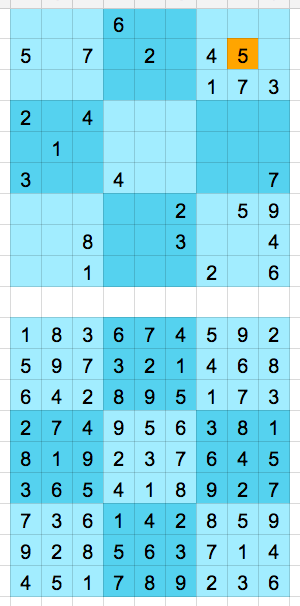Note: Since the Sudoku generator starts with a solution and removes numbers to make the puzzle, the Add-On could remember the solution and simply display it when the user presses "Solve". That's cheating. We don't cheat.

The Sudoku Sheets add-on uses Google's Linear Optimization Service to find solutions. Each cell in the grid is treated as a variable with nine boolean constraints: "is it 1?", "is it 2?", and so on. So in total there are 81 variables and 729 constraints.

Here's the relevant App Script code:

```
// Solves a Sudoku problem defined by input. if useRandomWeights is true, we create
// an objective function using random weights.
function solveFromInput(input) {
var solver = LinearOptimizationService.createEngine();
var size = input.length;
var blockSize = Math.sqrt(size);
if (blockSize * blockSize != size) {
throw 'Grid size must be the square of a number';
}
var width = input.length;
if (size != width) {
throw 'Grid must be a square';
}
// Variables
// cellVar(i,j,k) is a boolean variable, equal to 1 if grid cell (i,j) takes value k,
// 0 otherwise.
for (var i = 0; i < size; ++i) {
for (var j = 0; j < size; ++j) {
// Each cell can only take a single value:
// for all (i,j) in (rows, columns), Sum(k)cellVar(i,j,k) == 1
for (var k = 1; k <= size; ++k) {
// var(i,j,k) == 1 <=> cell(i,j) == k
if (input[i][j]) {
if (input[i][j] == k) {
solver.addVariable(cellVar(i, j, k), 1, 1, LinearOptimizationService.VariableType.INTEGER);
} else {
solver.addVariable(cellVar(i, j, k), 0, 0, LinearOptimizationService.VariableType.INTEGER);
}
} else {
solver.addVariable(cellVar(i, j, k), 0, 1,  LinearOptimizationService.VariableType.INTEGER);
}
uniqueValueCt.setCoefficient(cellVar(i, j, k), 1);
}
}
}

// Constraints: each value appears once one each row, column and sub-block.
for (var i = 0; i < size; ++i) {
for (var k = 1; k <= size; ++k) {
// Values appear once in each row:
// for all i in rows, k in values, Sum(j)cellVar(i,j,k) == 1
// Values appear once in each column:
// for all i in columns, k in values, Sum(j)cellVar(j,i,k) == 1
for (var j = 0; j < size; ++j) {
oncePerRowCt.setCoefficient(cellVar(i, j, k), 1);
oncePerColumnCt.setCoefficient(cellVar(j, i, k), 1);
}
}
}
for (var i = 0; i < blockSize; ++i) {
for (var j = 0; j < blockSize; ++j) {
for (var k = 1; k <= size; ++k) {
// Values appear once in each sub-block:
// for each block(i,j),
// Sum(blockRow,blockColumn,k)cellVar(i * blockSize + blockRow, j * blockSize + blockColumn, k) == 1
for (var blockRow = 0; blockRow < blockSize; ++blockRow) {
for (var blockColumn = 0; blockColumn < blockSize; ++blockColumn) {
oncePerBlockCt.setCoefficient(cellVar(i * blockSize + blockRow, j * blockSize + blockColumn, k), 1);
}
}
}
}
}
// Solve the Sudoku grid.
var solution = solver.solve(5);

var status = solution.getStatus();
if (status != LinearOptimizationService.Status.FEASIBLE && status != LinearOptimizationService.Status.OPTIMAL) {
// If the remote Linear Optim Service fails, try to solve locally.
if (status != LinearOptimizationService.Status.INFEASIBLE) {
return solveLocal(input);
}
return null;
}

// Output solution.
var out = [];
for (var i = 0; i < size; ++i) {
var row = []
for (var j = 0; j < size; ++j) {
for (var k = 1; k <= size; ++k) {
if (Math.round(solution.getVariableValue(cellVar(i, j, k))) == 1) {
row.push(k);
break;
}
}
}
out.push(row);
}
return out;
}
```